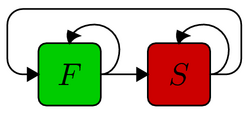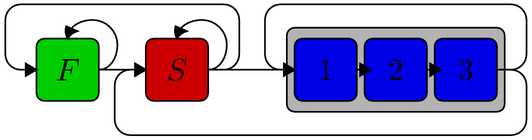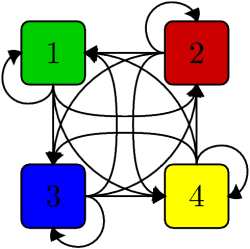# PRANK: structure models

PRANK can align sequences using a “structure model” that describes multiple evolutionary processes and infer regions evolving under each process along with the alignment. PRANK imports these alignment models from structured flat files but defining complex models manually can be an error-prone and frustrating task. The forms below provide a convenient way to build the most typical models for the alignment of DNA sequences, including the Two-state model describing regions of fast and slowly evolving sites and the Codon model describing the fast and slow regions and the periodicity of the three codon sites.

To build an alignment model using the forms below, modify the parameters as you wish and click the button in the bottom of the form. Depending on your browser, you will be directed to a new page or offered a text file to be saved. In either case, save the resulting page locally with a name that you will remember. You can then use this model for alignment by adding parameter -m=model_name in the PRANK command.

An alignment generated using the Two-state model (left) and the Codon model (right).

Description of a typical model file can be found in at the bottom of the page.

### Two-state modelThe simplest possible structure model consists of two distinct processes. As defined below, the model describes sequences with regions of fast and slowly evolving sites (F and S, respectively), such that the fast regions are, in average, slightly longer (length), have more gaps (gap rate) and the gaps tend to be longer (gap length). As long as the relative substitution rate is below 1, S is the slower process and the rate for F is adjusted such that the average rate equals 1. The base frequencies and the transition/transversion rate parameter kappa can be set separately for each process. Equal frequencies and kappa=1 correspond to the model of Jukes and Cantor.

F:

base freqs: A C G T    kappa

region length    gap rate    gap length

S:

base freqs: A C G T    kappa

region length    gap rate    gap length

relative substitution rate

### Codon modelCodon model is an extension of Two-state model with additional three processes depicting the three sites of a codon. As defined below, F, S and 1-3 describe regions of fast and slowly evolving sites and codons, respectively. The new parameters are the length of non-coding region (F + S) and, for codons, the intensity and direction of selection (omega) and the possible weighting of base frequencies according to empirical amino-acid frequencies (WAG). Small values of omega mean that the codons are under purifying selection and substitutions at the codon first and second sites are rare (generating the typical periodicity of “fast, fast, slow”), whereas omega=1 suggests that the sequences are evolving neutrally.

As coding sequence typically is preceded and followed by highly conserved splice regions and 1-3 can only be reached through S (see the figure), it is recommended that the relative substitution rate is below 1, making S the slower process.

F:
base freqs: A C G T    kappa

region length    gap rate    gap length

S:
base freqs: A C G T    kappa

region length    gap rate    gap length

relative substitution rate

length non-coding

1-3:

base freqs: A C G T

omega   WAG aa freqs

region length    gap rate    gap length

### Four-state modelFour-state model is a simple extension of the basic model and defines four separate single-character states with equal probabilities of moving from one state to any other state. The rate of the first state can not be defined but it is scaled such that the overall rate given the time spent in different states equals 1. See the description of Two-state model for more details.

1:

base freqs: A C G T    kappa

region length    gap rate    gap length

2:
base freqs: A C G T    kappa

length    gap rate    gap length

relative substitution rate

3:

base freqs: A C G T    kappa

region length    gap rate    gap length

relative substitution rate

4:

base freqs: A C G T    kappa

length    gap rate    gap length

relative substitution rate

### Format

Below is a two-state model generated using the default values. Comments (ignored by the program) are shown in red and the actual parameter values in black. Comments in blue are additional explanation and not found in a standard .hmm file.

```# Number of states   (obvious, isn't it? This is a two-state model!)
2
# Alphabet   (specifies DNA, RNA or protein -- or any other alphabet)
ACGT
# equilibrium distributions   (character frequencies for each state [1 and 2 here])
0.25 0.25 0.25 0.25
0.25 0.25 0.25 0.25
# Scaled Q matrices   (Q is instantaneous rate matrix, separately for each state)
# Model 1 ; scale = 0.855871   ('scale' can be seen as divider for branch length;
-1.1684 0.389467 0.389467 0.389467    as it is less than 1, branches are longer
0.389467 -1.1684 0.389467 0.389467    and sites are expected to evolve faster)
0.389467 0.389467 -1.1684 0.389467
0.389467 0.389467 0.389467 -1.1684
# Model 2 ; scale = 1.33333   ('scale' is greater than 1, so branches are shorter
-0.75 0.25 0.25 0.25            and sites are expected to evolve more slowly)
0.25 -0.75 0.25 0.25
0.25 0.25 -0.75 0.25
0.25 0.25 0.25 -0.75
# Structure background probabilities   (starting probability -- equal so no info)
0.5 0.5
# Structure transition probabilities   (probability to move from one structure to
0.9667 0.0333                         another or to stay in a structure [diagonal])
0.0500 0.9500
# Indel rates   (indel rates in the two structures; 2nd seems to have fewer indels)
0.0500 0.0250
# Indel extension probabilities   (indel lengths; 2nd seems to have shorter indels)
0.8000 0.5000
# Match extension probabilities   (for completeness; better kept 0?)
0 0
# Codon position   (two structures are non-coding; otherwise 1, 2 or 3)
0 0
```

### Acknowledgements

The code generating the alignment models relies heavily on Simon Whelan’s software multiscaleq.